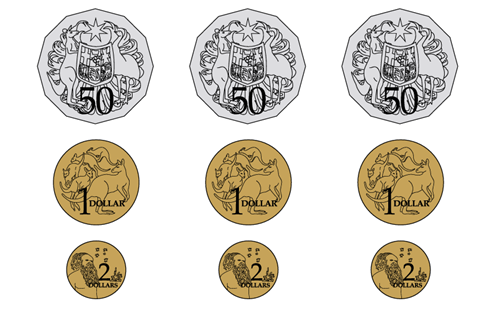# Understanding Australian coins

Students understand what Australian coins look like, their value and their purpose. They identify and describe the features and value of Australian coins and make simple calculations using coins.

Year level(s) Year 1, Year 2
Audience Teacher
Purpose Teaching resource, Teaching strategies
Teaching strategies and pedagogical approaches Questioning
Keywords coins, Australian money, financial literacy

## Curriculum alignment

Curriculum connections HASS, The Arts, Technologies
Strand and focus Number, Algebra
Topics Mathematical modelling, Money and financial mathematics
AC: Mathematics (V9.0) content descriptions
AC9M1A01
Recognise, continue and create pattern sequences, with numbers, symbols, shapes and objects, formed by skip counting, initially by twos, fives and tens

AC9M1N06
Use mathematical modelling to solve practical problems involving equal sharing and grouping; represent the situations with diagrams, physical and virtual materials, and use calculation strategies to solve the problem

AC9M2N04
Add and subtract one- and two-digit numbers, representing problems using number sentences and solve using part-part-whole reasoning and a variety of calculation strategies

Numeracy progression Counting processes (P6)
Number patterns and algebraic thinking (P3)
Multiplicative strategies (P2)